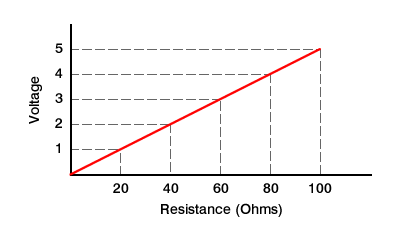I'm ordering components for my board, and I'm wondering what value would be appropriate for a linear pot. It will be used to take power from the 5V supply, and then pass to the analog in to convert to digital values.

I'm having trouble finding out how much current the 5V pin can output.

Because voltage is changing, current would remain constant through the pot (if I understand correctly).

Using V=IR I get the following. (I'm assuming a current of 50mA)

R=V/I

if V=0 and I=0.05A, then R=0
if V=5 and I=0.05A, then R=100

I made a graph to see if it would help me understand:This seems counterintuitive though, because if I were to pass the 5V source right to the analog in I believe that the resistance would be 0 (not accounting for the resistance of the wire). According to the formula 0 resistance should give me 0V.

If anyone can tell me what I'm doing horribly wrong it would be greatly appreciated. :-[

Connect one end of the potentiometer to 5V, the other end to ground, and the wiper to your analog input. Assuming you have a linear potentiometer, the voltage read by the ADC will gradually go from 0 to 1023 as you rotate the potentiometer from one end to the other.

If you want to know why, google resistor dividers.

You'll want to have a 10K to 50K potentiometer so you don't draw a lot of current.

I suppose my error was in assuming that a pot is some magical device that changes resistance when in fact it acts as 2 resistors in series. Thanks macegr.

This makes a lot more sense as I know in series the voltage across the input to the wiper and the wiper to ground must total 5.

When you came up with the values 10-50k, how were you able to know what the minimum/maximum current levels would be? This is more of a curiousity than anything else. I know enough to order the parts I need at least.

how were you able to know what the minimum/maximum current levels

The current through a pot is constant given by the pots value. That applies as long as you are not taking much current from the wiper. In this case you are not because the wiper goes to the analogue input of the arduino which is a very high resistance so it doesn't load the pot.

I think the question may be why 10k-50k. The answer to that is in the datasheet for the Arduino chip. Actually, the datasheet says the analog inputs are optimized for 10k or less so a 10k pot is probably the best choice.

I suppose my error was in assuming that a pot is some magical device that changes resistance when in fact it acts as 2 resistors in series.

Ackshewally, it's double magic, because you have two resistors changing their resistance ;D

When you came up with the values 10-50k, how were you able to know what the minimum/maximum current levels would be?

Almost all the current flows straight through the pot to ground, so you just use Ohm's Law, and divide the 5V by the resistance of the pot.

But that's almost all: the ADC input also draws a tiny amount of current. And you need to be careful that the ADC current remains tiny compared to the current flowing through the pot. If it gets relatively significant (i.e., if you use a high-value resistor), it will influence the A/D reading. So I prefer to use a pot on the order of 5-10K as a trade-off between low current and accuracy (and, btw, the ATMega datasheet says the ADC input is designed for signals with output impedance of 10K or less).

Ran

Actually, the datasheet says the analog inputs are optimized for 10k or less so a 10k pot is probably the best choice.

I'm looking through the datasheet for the ATMEGA 328P, and wondering what page you found this information on. I found a bunch of tables listing the electrical characteristics (pg. 313 onward), but am having trouble finding the value you referenced.

1 Like

Its in the Analog Input Circuitry section of the Analog-to-Digital converter chapter (section 21.6.1 on page 257 of the ATmega168/328 datasheet Rev. 80251-AVR-02/09)

The second paragraph begins: “The ADC is optimized for analog signals with an output impedance of approximately 10 k[ch937] or less.”

1 Like